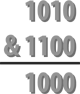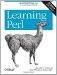# Section 11.4. Bitwise Operators

### 11.4. Bitwise Operators

When you need to work with numbers bit by bit, as when working with the mode bits returned by stat, you'll need to use the bitwise operators. These operators perform binary math operations on values. The bitwise-and operator (&) reports which bits are set in the left argument and in the right argument. For example, the expression 10 & 12 has the value 8. The bitwise-and needs to have a one-bit in both operands to produce a one-bit in the result. That means that the logical-and operation on ten (which is 1010 in binary) and twelve (which is 1100) gives eight (which is 1000, with a one-bit only where the left operand has a one-bit and the right operand also has a one-bit). See Figure 11-1.The different bitwise operators and their meanings are shown in Table 11-2.

##### Table 11-2. Bitwise operators

Expression

Meaning

10 & 12

Bitwise-and; which bits are true in both operands (this gives 8)

10 | 12

Bitwise-or; which bits are true in one operand or the other (this gives 14)

10 ^ 12

Bitwise-xor; which bits are true in one operand or the other but not both (this gives 6)

6 << 2

Bitwise shift left; shift the left operand the number of bits shown by the right operand, adding zero-bits at the least-significant places (this gives 24)

25 >> 2

Bitwise shift right; shift the left operand the number of bits shown by the right operand, discarding the least-significant bits (this gives 6)

~ 10

Bitwise negation, also called unary bit complement; return the number with the opposite bit for each bit in the operand (this gives 0xFFFFFFF5, but see the text)

So, here's an example of some things you could do with the \$mode returned by stat. The results of these bit manipulations could be useful with chmod, which you'll see in Chapter 12:

`     # \$mode is the mode value returned from a stat of CONFIG     warn "Hey, the configuration file is world-writable!\n"       if \$mode & 0002;                                # configuration security problem     my \$classical_mode = 0777 & \$mode;                # mask off extra high-bits     my \$u_plus_x = \$classical_mode | 0100;            # turn one bit on     my \$go_minus_r = \$classical_mode & (~ 0044);      # turn two bits off `

#### 11.4.1. Using Bitstrings

All of the bitwise operators can work with bitstrings, as well as with integers. If the operands are integers, the result will be an integer. (The integer will be at least a 32-bit integer but may be larger if your machine supports that. That is, if you have a 64-bit machine, ~10 may give the 64-bit result 0xFFFFFFFFFFFFFFF5, rather than the 32-bit result 0xFFFFFFF5.)

But if any operand of a bitwise operator is a string, Perl will perform the operation on that bitstring. That is, "\xAA" | "\x55" will give the string "\xFF". Note that these values are single-byte strings and the result is a byte with all eight bits set. Bitstrings may be arbitrarily long.

This is one of the few places where Perl distinguishes between strings and numbers. See the perlop manpage for more information on using bitwise operators on strings.Learning Perl, 5th Edition
ISBN: 0596520107
EAN: 2147483647
Year: 2003
Pages: 232

Similar book on Amazon